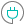#LOGNORMDIST (PQL - xl)

This function returns the cumulative natural log-normal distribution of x, where LN(x) is normally distributed with the specified mean and standard deviation. Analyze data that has been logarithmically transformed with this function

#### Syntax

LOGNORMDIST(x, mean, standard dev)

##### Function Arguments
 Name Description Type Optional x Value at which to evaluate the function Number mean Value of mean of the natural logarithm of x, LN(x) Number standard dev Value representing the standard deviation of LN(x) Number